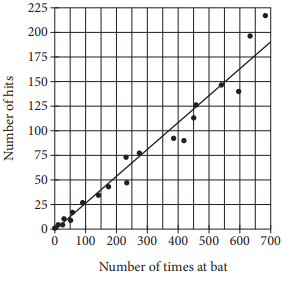mycollegehive
The Number of Hits and Times at Bat by Players on a Major Le...
PSAT PSAT-math-test math0The Number of Hits and Times at Bat by Players on a Major League Baseball TeamThe scatterplot above shows the number of hits and the number of times at bat by each of 20 players on a major league baseball team. The line of best fit for the data is also shown.

Which of the following statements about the relationship between the number of times at bat and the number of hits is true?

A) As the number of times at bat increases, the number of hits decreases.

B) As the number of times at bat increases, the number of hits increases.

C) As the number of times at bat increases, the number of hits remains constant.

D) As the number of times at bat decreases, the number of hits increases.

119 viewsShareFollowSchool not set Nigeria
06 January 2021University of Benin Nigeria
06 January 20210The line of best fit shown for the data has a positive slope. It can be concluded from this that higher values for the number of times at bat correspond to higher values for the number of hits. Therefore, as the number of times at bat increases, the number of hits increases.Share

### Groups

How to insert math formulas/equations### Related Tags

PSAT

3 followers

94 questionsPSAT-math-test

4 followers

94 questionsmath

23 followers

1262 questions